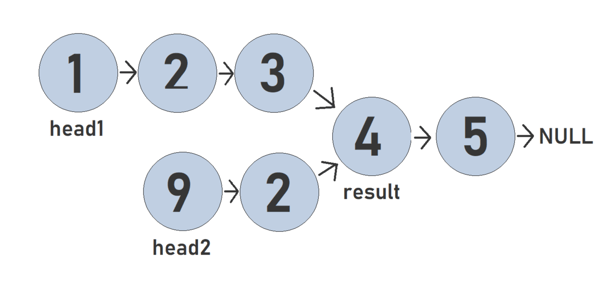Related Tags

communitycreator

# How to find the merge point for two linked lists

Dhruv Sharma

### Overview

A linked list is a collection of nodes. Each node contains data and a pointer to the next node.

A linked list is preferred over other data structures like arrays because it has a dynamic size and it's easier to insert and delete elements/nodes.

class Node:
def __init__(self, data):
self.data = data
self.next = None

def __init__(self):
self.tail = None

def print_ll(self):
while temp:
print(str(temp.data))
temp = temp.next

def insert(self, data):
node = Node(data)
else:
self.tail.next = node
self.tail = node

l1val = [1,2,3,4,5]

for i in l1val:
l1.insert(i)

l1.print_ll()

### Explanation

In a linked list, the head attribute refers to the first node of a list. All the functions used in the list are added in the SinglyLinkedList class.

Node class is used to create a new node, each node has a data attribute and a pointer attribute to the next node which initially points to a null.

### Understanding the Problem

Consider two linked lists that will meet at some point. The head nodes of both lists are given pointers to find the node where the two lists merge.Creating the merge for the linked lists

### Example

In the above example, the two lists merge at Node 4, which is the result of the function.

### Solution

In the program below, we use the merge_point() function to find the data of the node where both the lists merge.

class SinglyLinkedListNode:
def __init__(self, data):
self.data = data
self.next = None

def __init__(self):
self.tail = None

def insert(self, data):
else:
self.tail.next = node
self.tail = node

data = []

l1val = [1,2,3,4,5]
l1_count = 5
for i in l1val:
l1.insert(i)

l2val = [9,2]
l2_count = 2
for i in l2val:
l2.insert(i)

for i in range(l1_count):
if i < 3:
ptr1 = ptr1.next

for i in range(l2_count):
if i != l2_count-1:
ptr2 = ptr2.next
ptr2.next = ptr1


Finding merge point of two linked lists

### Explanation

We follow the steps below to run the merge_point() function:

Lines 21–23: Traverse the first linked list and add all the nodes in a list called data

Lines 24–25: Check if any node exists in the data list to traverse the second linked list.

Lines 25–26: Use an exit condition to return the data of the node which exist in the data list.

RELATED TAGS

communitycreator

CONTRIBUTOR

Dhruv Sharma
RELATED COURSES

View all Courses

Keep Exploring

Learn in-demand tech skills in half the time